International Journal of Computational and Theoretical Chemistry
Volume 3, Issue 4, July 2015, Pages: 28-33

Fourth Order Virial Equation of State of a Nonadditive Lennard - Jones Fluid

Kenneth Osondu Monago1, Charles Otobrise2, *

1Department of Pure and Industrial Chemistry, University of Port - Harcourt, Choba, Port - Harcourt, Nigeria

2Department of Chemistry, Delta State University, Abraka, Nigeria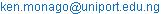(K.O. Monago)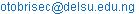(C. Otobrise)

Kenneth Osondu Monago, Charles Otobrise. Fourth Order Virial Equation of State of a Nonadditive Lennard - Jones Fluid. International Journal of Computational and Theoretical Chemistry. Vol. 3, No. 4, 2015, pp. 28-33. doi: 10.11648/j.ijctc.20150304.11

Abstract: A fourth - order virial equation of state was combined with the Lennard – Jones potential and the Axilrod - Teller triple - dipole potential to determine the thermodynamic properties of argon in the gas phase. The fourth virial coefficient is exact at the level of graphs with at most three non - additive three - body potentials. The model parameters were determined in a fit to the speed - of - sound data. The equation of state predicted the second (volumetric and acoustic) and the fourth acoustic virial coefficients of argon, but failed to give quantitative predictions of the third (volumetric and acoustic) and the fourth volumetric virial coefficients. For the third and fourth volumetric virial coefficients in which the equation of state failed to provide quantitative predictions, it nevertheless provided qualitatively accurate information on the variation of thesefunctions with temperature.In the region of the critical point, the model can be used for exploratory calculations at densities up to about 0.9ρc.

Keywords: Lennard - Jones Potential, Volumetric Virial Coefficients of Argon, Acoustic Virial Coefficients of Argon, Fourth Virial Coefficients of Argon, Axilrod - Teller Triple - Dipole Potential

1. Introduction

The Lennard - Jones (12 - 6) potential, Eq. (1), is the simplest function that accounts for the shape of interatomic potential in the short, medium and long range regions of interatomic separation.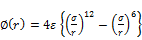(1)

The attractive part of the potential is the leading dipole - dipole dispersion energy that arises in the second - order of the Rayleigh - Schrodinger perturbation theory ; the repulsive partis purely empirical. Although partly based on theory, it has long been recognized that the 12 - 6 functional form has several deficiencies, which include; (a) the well - depth parameter as obtained from fitting to bulk properties is smaller than in real potentials and, (b) at large separations, the function decays too slowly, leading to a C6 coefficient that is overestimated by some 80 percent. However, despite its deficiencies the 12 - 6 function remains one of the most used potentials, especially in computer simulation studies where it has found extensive use. The reasons for its popularity may be its mathematically simple form and that it does not predict physical absurdity. Moreover, it is desirable to keep the number of model parameters to a minimum at the pure fluid level so that the number of parameters does not become unmanageablewhen one proceeds to mixtures. The simplicity of the function is important in computationally demanding applications; for example, it takes about 16 hours to calculate the third virial coefficient (volumetric plus acoustic) for a real, symmetric linear molecule with the Maitland - Smith potential, but only 8 hours if one uses the 12 - 6 potential.

In computer simulation, the Lennard - Jones model fluid is defined as one that interacts according to Eq. (1) and, two, the N - body potential is pairwise additive. The concept of a model fluid is useful because it offers an unambiguous standard against which statistical mechanical theories can be tested. However, in general, the N - body potential is not pairwise additive in real fluids and there are nonadditive contributions to the total potential from clusters of three or more molecules. Barker showed that, using an accurate pair - potential, calculated third volumetric virial coefficients of argon did not agree with experimental values unless nonadditivetriple - dipole contributions were included . Unfortunately, there are few published simulation studies that include non - additive three - body forces and the few that exist pertains only to the third virial coefficient [3,4]. Therefore, thermodynamic properties that are derived from the Lennard - Jones fluid cannot be compared to the data of real fluids in any meaningful way.

Recent works haveshown that a fourth - order virial equation of state predicted the P - V - T properties of argon to within an accuracy of 0.05 percent at densities up torc/2, or in pressure up to 12 MPa [5,6]. In ref. 5, the equation of state was based on the Maitland - Smith potential function and non - additive interaction was modelled by the Axilrod - Teller potential. The purpose of this work is, therefore, to use the 12 - 6 potential and the Axilrod - Teller triple dipole potential to implement a fourth - order virial equation of state and to compare interesting derived thermodynamic properties from this model with experimental data and the equation of state from the method of ref. 5.

2. Theoretical Approach

The Lennard - Jones 12 - 6 potential contains two parameters: the well - depth parameter, e, and hard - core parameter, s. In this work, the two parameters were determined by a fit to the speed - of - sound data of Estrada - Alexanders and Trusler, . The basic physical equations that relate equilibrium speed of sound, w, to other thermodynamic properties may be set out as follows :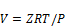(2)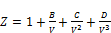(3)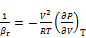(4)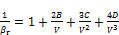(5)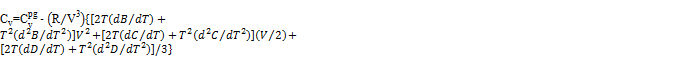(6)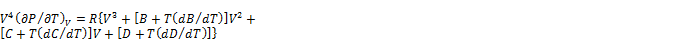(7)(8)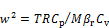(9)

In Eq. (2) – (9), Z is the compressibility factor and B, C, D are, respectively, the second, third and fourth volumetric virial coefficients, which are functions only of temperature. The other quantities have their usual meanings.

The volumetric virial coefficients are related to the potentials of interaction by integral expressions; in the language of linear graph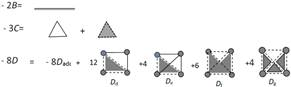(10)

In Eq. (10), an unbroken line represents the two - body Mayer function, a dashed line represents the Boltzmann factor and a shaded triangular plate represents the three - body Mayer function. Explicit expressions for all the graphs, except for Dg, have been given elsewhere, [8,9].

In Eq. (3) the expansion of compressibility factor in powers of density assumes that the total intermolecular potential energy f (rN) is the sum of pair and triplet interactions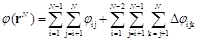(11)

Where, fij is the pair - potential energy and Dfijk is the non - additive three - body potential energy of interaction. Furthermore, in Eq. (10) whereas the graph prescriptions for B and Care exact, the prescription for Dis exact only at the level of graphs with no more than three triplet potentials; the graph with four triplet potentials and the non - additive four - body potential have been neglected. The computational integral expression for the Dg graph is: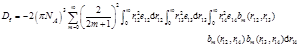(12)

All the quantities in Eq. (12) are as defined in previous works, [8,9].Non - additive three - body forces are modeled by the Axilrod - Teller triple dipole energy, Eq. (13)(13)

Eq. (13) adds the third parameter to the molecular model namely, J123, the three - body strength coefficient.

When experimentalists analyse experimental speed - of - sound isotherms of gaseous substances, the preferred methodology is not the procedure outlined above, rather acoustic data along an isotherm are expanded in powers of density as in Eq. (14)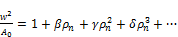(14)

Where, rn is the amount - of - substance density, b, g and d are, respectively, the second, third and fourth acoustic virial coefficients. Acoustic virial coefficients, like their volumetric counterparts, are functions only of temperature. The quantity A0 is defined by Eq. (15)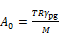(15)

In Eq. (15), R is the molar gas constant, M is the molar mass and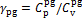; where,and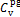are, respectively, the isobaric and isochoric heat capacity of the hypothetical perfect - gas.

Volumetric and acoustic virial coefficients are not independent, but are related as set out in Eq. (16) – (18) below: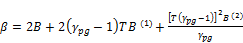(16)(17)(18)

In Eq. (16) – (18), X(1) represents dX/dT and X(2) represents d2X/dT2; where, X is one of the volumetric virial coefficients, B, C orD, [10,11].

3. Numerical Methods

The three parameters in the molecular model were determined by solving the non - linear least square problem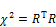(19)

as explained in a previous publication . In Eq. (19),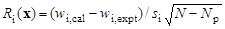(20)

and x is the parameter vector, wi, expt is the i - th experimental speed of sound value, wi, cal is the calculated value for the speed of sound at the i - th data point, N is the total number of acoustic data points, Np is the number of adjustable parameters in the model and si is the estimated standard deviation of wi, expt.

Parameter optimisation using the systems of equations (2) - (9) and (19) was performed as described in previous works, [8,9]. The second virial coefficient, B, and its two temperature derivatives were calculated as described in ref. 9. Numerical integration of C and its temperature derivatives were truncated beyond r=20rm, the range of integration was divided into 40 equal panels each of which was evaluated by an eleven - point Gaussian quadrature. The graphs in D (including Dg) and their temperature derivatives were also calculated as described in ref. 9; except that each graph was assumed to be zero beyond r=8rm and for each integral the range was divided into 16 equal panels each of which was evaluated by an eleven - point Gaussian quadrature.

4. Results and Discussion

The values (in reduced units) of the graph Dg are tabulated in table 1 at selected reduced temperatures for a Lennard - Jones potential.

Table 1. Values of the graph.

 T* T* 0.6 - 0.05513 1.2 - 0.00023 0.8 - 0.00394 1.4 - 0.00014 1.0 - 0.00074 1.5 - 0.00009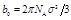,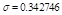nm,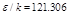K,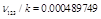Knm9, T* = kT/e.

4.1. The Fit to the Speed of Sound

The experimental speed - of - sound data on argon performed by Estrada - Alexanders,were given on 17 isotherms in the region 0.72£T/Tc£1.47 and r£rc/2; all the experimental isotherms were used in the parameter fitting. However, on each isotherm we limited acoustic data employed in the fit to those at densities that were no higher than 1.03 moldm-3 and each datum was assigned an uncertainty of 0.003 percent. The following set of parameters minimized Eq. (19):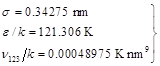(21)

4.2. Potential Parameters

The potential parameters obtained in this work (Eq. 21) were compared with current best theoretical estimates: LJP, model based on the Lennard - Jones potential (this work); MSP, model based on the Maitland - Smith potential . The references in the table refer to the theoretical estimates.

Table 2. Potential Parameters Determined in Fits toAcoustic Data.

 Potentialparameter Percentage error Ref. LJP MSP rm 2. - 0.1  e/k - 15 0.5  C6/k 76 - 8  J123/k - 4 4 

4.3. The Virial Coefficients of Argon

Fig. 1 compares the experimental second volumetric virial coefficients of Gilgen et al.,with results obtained from the present work and the obvious conclusion is that, in spite of the wide temperature range, the 12 - 6 potential is able to correlate the data. However, as fig. 2 shows, with the 12 - 6 function deviations in calculated second virial coefficient can exceed2cm3/mol, which may be compared with a maximum discrepancy of about 0.4 cm3/mol obtained with the Maitland - Smith potential. In fig. 3 we compare the experimental second acoustic virial coefficient data of Estrada - Alexanders and Trusler , with values obtained from the present work; the agreement is good. Indeed, as the deviation plotin fig. 4 shows, both volumetric and acoustic virial coefficients are correlated with nearly comparable levels of accuracies. As fig. 1 and 3 have shown, the two parameters in the 12 - 6 potential can be chosen to give a reasonably good representation of the second (volumetric and acoustic)virial coefficients of argon; however, when the pair - potential is combined with the triple - dipole potential, it appears that the pair - potential is not sufficiently flexible to determine the three - body strength coefficient. The result is that the 12 - 6 function fails to correlate, quantitatively, the third virial coefficient of argon, as fig. 5 and 6 show. Similarly, the 12 - 6 potential when combined with the triple - dipole potential is unable to accurately represent the fourth volumetric virial coefficient of argon, as fig. 7 also shows. However, in all cases, the 12 - 6 potential does provide qualitatively correct representation of the properties concerned.

Considering that the 12-6 potential failed to correlate the third (volumetric and acoustic) virial coefficients and the fourth volumetric virial coefficients, the situation displayed in fig. 8 is a rather curious one. The plausible explanation is that the apparently good representation achieved in that plot was the result of fortuitous cancellation of errors in the other virial coefficients and their temperature derivatives.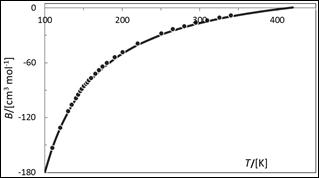Fig. 1. Second volumetric virial coefficient of argon as afunction of temperature: ● experimental data from Vrabec et al., (2001); —— values calculated from Lennard - Jones (12 - 6) potential function.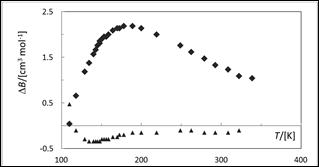Fig. 2. Deviations DB = Bexpt–Bcal of experimental second volumetric virial coefficient of argon from values calculated from different pair - potential functions: ▲, Bcal is from the Maitland - Smith potential function; ♦, Bcalis from the 12 - 6 potential function.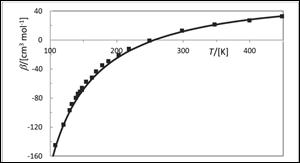Fig. 3. Second acoustic virial coefficient of argon as a function of temperature: ■ values from Estrada - Alexander and Trusler, (1995); ——, values calculated from the 12 - 6 potential function.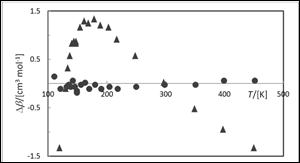Fig. 4. Deviations Db = bexptbcal of experimental second acoustic virial coefficient of argon from values calculated from different pair - potential functions: ●, bcal is from the Maitland - Smith potential function; ▲, bcalis from the 12 - 6 potential function.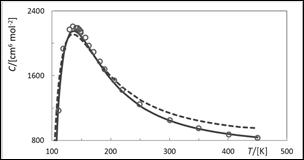Fig. 5. Third volumetric virial coefficient of argon as a function of temperature: ▲ experimental data from Estrada - Alexander and Trusler, (1995); ———, values calculated from the Maitland - Smith potential function; - - -  - -, values calculated from the 12 - 6 potential function.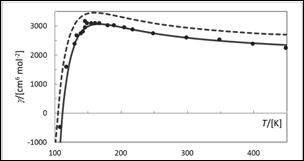Fig. 6. Third volumetric virial coefficient of argon as a function of temperature: ● experimental data from Estrada - Alexander and Trusler, (1995); ———, values calculated from the Maitland - Smith potential function; - - -, values calculated from the 12 - 6 potential function.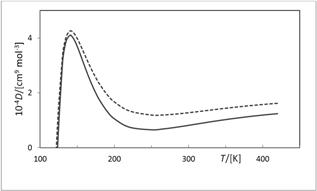Fig. 7. Fourth volumetric virial coefficient of argon as a function of temperature: ———, values calculated from the Maitland - Smith potential; - - - - - - - - - - -, values calculated from the 12 - 6 potential function.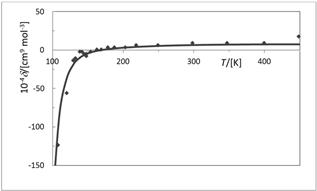Fig. 8. Fourth acoustic virial coefficient of argon as a function of temperature: ♦, experimental data from Estrada - Alexanders (1995); —————, values calculated from the 12 - 6 potential function.

4.4. Comparison with Other Experimental Data

It is the aim of applied statistical thermodynamics to generate molecular models that relate macroscopic properties to their underlying causes in intermolecular interaction . Although the virial equation of state, because it is inapplicable at liquid densities, is not suitable for vapour - liquid equilibrium calculations, given experimental vapour pressure data it can be used to calculate the vapour densities at saturation. Fig. 9 shows such calculations for the experimental vapour pressure data of argon .It shows that the 12 - 6 potential can predict saturated vapour densities with reasonably good accuracy up to aboutrc/2. Furthermore, one may use the 12 - 6 function for exploratory calculations up to about 0.8rc. Another area of process thermodynamics in which the virial equation has found use is in the field of supercritical fluids extraction. In fig. 10 we show the performance of the current equation of state model when it is used to calculate fluid densities along the critical isotherm (150.69 K). The figure shows that the current equation of state can provide useful volumetric data on argon up to a density of about 0.9rc. Of course the present model being analytic cannot be expected to reproduce the actual vapour - liquid critical point itself for argon, or for any other fluid for that matter .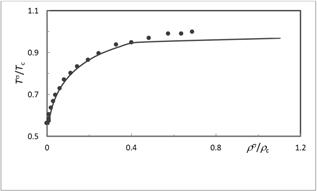Fig. 9. Reduced Vapour density of argon as a function of reduced temperature: ●, experimental data from ref. 14; —————, values calculated from the 12 - 6 potential. The superscript s denotes saturation.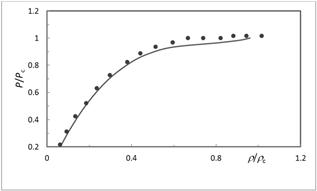Fig. 10. Reduced pressure of argon along the critical temperature as a function of reduced density: ●, experimental data from ref. 14; —————, values calculated from the 12 - 6 potential.

5. Conclusion

In this work, the Lennard - Jones pair potential was combined with the Axilrod - Teller triple dipole potential to derive a fourth - order virial equation of state. The fourth virial coefficient is exact at the level of graphs with at most three triplet potentials andthe method was applied to argon in the gas phase. The model predicted reasonably accurate values for the second volumetric and acoustic virial coefficients and the fourth acoustic virial coefficient, but failed to predict quantitatively accurate third volumetric and acoustic virial coefficients and the fourth volumetric virial coefficient. However, for those coefficients that the model failed to predict quantitatively, it nevertheless predicted their values as functions of temperature, which were qualitatively accurate.We investigated the performance of the virial equation of state in the region of the critical point and found that the model can be used for exploratory calculations at densities up to about 0.9rc.

References

1. R. J. Bell and I. J. Zucker, (9176), in "Rare gas Solids Vol. 1", M. L. Klein and J. A. Venables (eds.), Academic Press, pp 123 – 175.
2. J. A. Barker, Mol. Phys., (1986), 57 (4), 755 – 760.
3. A. J. Masters, J. (2008), Phys. Condens. Matter, 20, 1 – 10.
4. P. G Kusalik, F. Liden and I. M. Svishchev, (1995), J. Chem. Phys. B, 103, 10169 – 10175.
5. K. O. Monago, (2013), Chem. Phys., 441, 45 – 48.
6. J. Wiebke, P. Scherdtfeger, G. E. Moyano, E. Pahl, (2011), Chem. Phys. Lett., 514, 164 – 167.
7. A. F Estrada - Alexanders and J. P. M. Trusler, (1995), J. Chem. Thermodyn., 27, 1075 – 1089.
8. K. O. Monago, (2007), Chem. Phys., 337, 125 – 134.
9. K. O. Monago, (2010), Korean J. Chem. Eng., 27, 590 – 595.
10. A. F. Estrada - Alexanders, (1995), Ph.D. thesis, University of London.
11. W. Van Dael, (1975), in "Experimental Thermodynamics vol. 2: Experimental Thermodynamics of Non - reacting Fluids", B. Le Neindre and B. Vodar (eds.), Butterworths, London, pp 527 – 574.
12. S. J. Boyes, (1994), Chem. Phys. Lett., 221, 467 – 472.
13. A. Kumar and W. J. Meath, (1985), Mol. Phys., 54, 823–833.
14. R. Gilgen, R. Kleinrahm and W. Wagner, (1994), J ChemThermodyn., 26, 384 – 398.
15. J. Vrabec, J. Stoll and H. Hasse, (2001), J. Phys. Chem. B, 105, 12126 – 12133.
16. R. Gilgen, R. Kleinrahm and W. Wagner, (1994), J ChemThermodyn., 26, 399 – 413.
17. K. E. Bett, J. S. Rowlinson and G. Saville, (2003), "Thermodynamics for Chemical Engineers", Athlone Press, London, pp 148 – 150.

 Contents 1. 2. 3. 4. 4.1. 4.2. 4.3. 4.4. 5.
Article ToolsAbstractPDF(1048K)